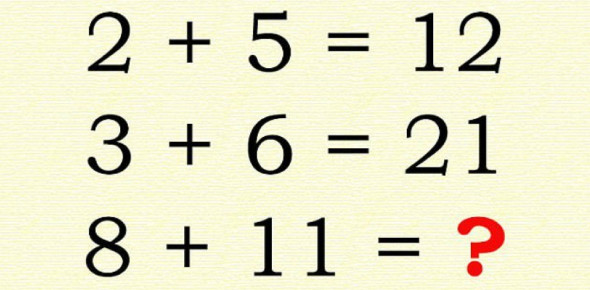# Unlock The World Of Numbers: Basic Math Problems Quiz

Reviewed by Janaisa Harris
Janaisa Harris, BA-Mathematics |
Mathematics Expert
Review Board Member
Ms. Janaisa Harris, an experienced educator, has devoted 4 years to teaching high school math and 6 years to tutoring. She is now broadening her educational impact by engaging in curriculum mapping for her county. This endeavor enriches her understanding of educational strategies and their implementation.
, BA-Mathematics
Approved & Edited by ProProfs Editorial Team
The editorial team at ProProfs Quizzes consists of a select group of subject experts, trivia writers, and quiz masters who have authored over 10,000 quizzes taken by more than 100 million users. This team includes our in-house seasoned quiz moderators and subject matter experts. Our editorial experts, spread across the world, are rigorously trained using our comprehensive guidelines to ensure that you receive the highest quality quizzes.
| By Loulau
L
Loulau
Community Contributor
Quizzes Created: 1 | Total Attempts: 64,980
Questions: 10 | Attempts: 67,001SettingsIs your child aged 6-10 and eager to explore the world of math? Look no further! Our Basic Math Problems Quiz is tailor-made for primary school children at the early stages of their mathematical journey. Problem-solving is a critical skill in a child's development, and this quiz is here to nurture that.
Designed with age-appropriate questions, this quiz offers a fun and educational way for young minds to practice basic math. Your child will face a series of math problems, providing opportunities to apply their knowledge and develop problem-solving abilities.
Encourage your child to tackle as many questions Read moreas possible and aim for a perfect score. It's not just a quiz; it's a chance for them to build confidence and proficiency in math. Best of luck to your young mathematician on their quest for excellence!

• 1.

### Which answer choice represents the equal sign symbol?

• A.

?

• B.

+

• C.

=

• D.

-

C. =
Explanation
The equal sign (=) is a mathematical symbol used to indicate that two values or expressions are equal. In this context, the question is asking for the definition or meaning of the equal sign.

Rate this question:

• 2.

### What's the sign of multiplication?

• A.

X

• B.

?

• C.

-

• D.

+

A. X
Explanation
The sign of multiplication is represented by the symbol "x". This symbol indicates that two or more numbers are being multiplied together. It is commonly used in mathematical equations and represents the operation of multiplication.

Rate this question:

• 3.

### What is 42 divided by 14?

• A.

3

• B.

4

• C.

6

• D.

7

A. 3
Explanation
The correct answer is 3 because when you divide 42 by 14, you get 3 as the quotient.

Rate this question:

• 4.

### If a person has 440 pens and 560 pencils, how many more pencils does he have than pens?

• A.

110

• B.

120

• C.

130

• D.

140

B. 120
Explanation
The person has 560 pencils and 440 pens. To find out how many more pencils he has, we subtract the number of pens from the number of pencils. 560 - 440 = 120. Therefore, the person has 120 more pencils.

Rate this question:

• 5.

### What's 6 multiplied by 6?

• A.

33

• B.

34

• C.

35

• D.

36

D. 36
Explanation
The correct answer is 36 because when you multiply 6 by 6, you get the product of 36.

Rate this question:

• 6.

### What's 8 - 3.5?

• A.

2.5

• B.

4.5

• C.

5.5

• D.

6.5

B. 4.5
Explanation
The correct answer is 4.5 because when you subtract 3.5 from 8, you get 4.5.

Rate this question:

• 7.

### 7 + 8 + 9 + 10 =

• A.

34

• B.

36

• C.

38

• D.

40

A. 34
Explanation
The correct answer is 34 because when you add 7, 8, 9, and 10 together, the sum is 34.

Rate this question:

• 8.

### How much is the total of 667 oranges and 301 bananas?

• A.

801

• B.

968

• C.

998

• D.

979

B. 968
Explanation
The correct answer is 968 because it is the sum of 667 oranges and 301 bananas.

Rate this question:

• 9.

### What's 472 multiplied by 0?

• A.

529

• B.

17

• C.

782

• D.

0

D. 0
Explanation
When any number is multiplied by 0, the result is always 0. In this case, 472 multiplied by 0 will also be 0. This is because when we multiply any number by 0, we are essentially adding zero copies of that number together, resulting in a total of 0. Therefore, the correct answer is 0.

Rate this question:

• 10.

### Which of these answer choices produces the biggest value?

• A.

450 - 78

• B.

12 x 12

• C.

700 - 301

• D.

258 + 107

C. 700 - 301
Explanation
The biggest number among the given options is determined by subtracting 301 from 700.

Rate this question:

Janaisa Harris |BA-Mathematics |
Mathematics Expert
Ms. Janaisa Harris, an experienced educator, has devoted 4 years to teaching high school math and 6 years to tutoring. She is now broadening her educational impact by engaging in curriculum mapping for her county. This endeavor enriches her understanding of educational strategies and their implementation.

Related TopicsBack to top